Bitcoin Forum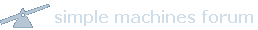January 25, 2020, 07:23:19 PM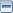News: Latest Bitcoin Core release: 0.19.0.1 [Torrent]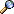Home Help Search Login Register More
 Pages:Author Topic: How is a public key calculated from a private key using ecc?  (Read 147 times)
Anonymous Kid
Member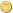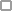Offline

Activity: 183
Merit: 23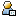October 02, 2018, 07:39:21 AMMerited by theymos (4), bones261 (2)

As I understand it a pub key is calculated by;

u = random number (private key)
(x, y) = G = Base point

uG = P = public key

my question is, if its possible to calculate the pub key with uG then since you are doing u computations already, can't you just calculate the private key from P?
Just brute force G as many times as needed until you arrive at P.

Obviously I am misunderstanding something. Is P calculated in a more efficient way than just u*G?15799801991579980199

1579980199
 Report to moderator
Advertised sites are not endorsed by the Bitcoin Forum. They may be unsafe, untrustworthy, or illegal in your jurisdiction. Advertise here.
15799801991579980199

1579980199
 Report to moderator
tromp
Hero MemberOffline

Activity: 627
Merit: 551October 02, 2018, 08:03:58 AMMerited by theymos (4), bones261 (2), ETFbitcoin (1), Anonymous Kid (1)

> Obviously I am misunderstanding something. Is P calculated in a more efficient way than just u*G?

Yes; u*G is computed in time logarithmic in u, not linear in u as you seem to think.
For instance 13*G is computed as 2 * (2 * (2 * G + G)) + G.Anonymous Kid
MemberOffline

Activity: 183
Merit: 23October 02, 2018, 08:27:33 AM

Yes; u*G is computed in time logarithmic in u, not linear in u as you seem to think.
For instance 13*G is computed as 2 * (2 * (2 * G + G)) + G.

Ah yes. This makes a lot more sense. Thanks.aplistir
Full MemberOffline

Activity: 366
Merit: 170October 02, 2018, 12:57:23 PMLast edit: October 02, 2018, 01:51:49 PM by aplistir

my question is, if its possible to calculate the pub key with uG then since you are doing u computations already, can't you just calculate the private key from P?
Just brute force G as many times as needed until you arrive at P.

The numbers are so big that brute forcing is not possible with the technology that we currently have. Even if all the existing computers would be used simultaneously for only that purpose, it would still take more than 10000 years to brute force ONE bitcoin key. (Much, much more than 10000 years.)

Yes; u*G is computed in time logarithmic in u, not linear in u as you seem to think.
For instance 13*G is computed as 2 * (2 * (2 * G + G)) + G.

Correct, but I think the same can be more clearly explained in the way  it is actually done:
First pre-calculate the values:
G, 2*G, 4*G, 8*G, 16*G, 32*G ....... 2²⁵⁶*G

Then these pre-calculated values are used for getting any desired value by using only addition.
eg:
13*G= 8*G+4*G+G
This is faster, because you do not have to do the same multiplications every time. You can just use addition. And to do this you only need to keep the 256 precalculated values in memory.andytoshi
Full MemberOffline

Activity: 174
Merit: 132

-October 02, 2018, 02:03:25 PMMerited by achow101 (3), TheArchaeologist (3), ETFbitcoin (1)

Not to detract from the main point, but if you were implementing this multiplication yourself, using the "powers of two" ladder is a bad idea for security reasons. Specifically, for each 1 bit in your secret key you're adding 2^i*G, while for each 0 bit you're not doing anything. Somebody timing your algorithm could easily determine how many powers of 2 you added, i.e. how many bits in your secret key are 1.

In general, you want to use a multiplication algorithm that never puts secret data into if-conditionals  or into array indices . It is possible to efficiently do this, which I think is actually even more surprising than the fact that you can do the multiplication in sublinear time.

One such algorithm, though by no means the most efficient one, is as follows (a special case of , unless I screwed it up):

1. Compute x' = (x + 2^256 - 1)/2 mod the secp256k1 curve order. The bits of this number have a special property: if you interpret all the 0's as -1's, they also represent the original secret key x. See , top of page 9.
2. Compute G, -G, 2G, -2G, 4G, -4G, ..., 2^256G, -2^256G and put them in an array P.
3. Compute the sum of { x_i'*P[2*i + 1] + (1 - x_i')*P[2*i] } over each bit x'_i of x'.

 "Don't branch on secret data" is folklore, I don't have a citation for it.
 https://cryptojedi.org/peter/data/chesrump-20130822.pdf
 http://www.tau.ac.il/~tromer/papers/cache.pdf
 https://github.com/bitcoin-core/secp256k1/pull/546
 https://eprint.iacr.org/2012/309.pdfPages: